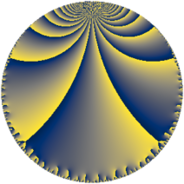# Properties

 Label 80.6.lLevel $80$ Weight $6$ Character orbit 80.l Rep. character $\chi_{80}(21,\cdot)$ Character field $\Q(\zeta_{4})$ Dimension $80$ Newform subspaces $1$ Sturm bound $72$ Trace bound $0$

# Related objects

## Defining parameters

 Level: $$N$$ $$=$$ $$80 = 2^{4} \cdot 5$$ Weight: $$k$$ $$=$$ $$6$$ Character orbit: $$[\chi]$$ $$=$$ 80.l (of order $$4$$ and degree $$2$$) Character conductor: $$\operatorname{cond}(\chi)$$ $$=$$ $$16$$ Character field: $$\Q(i)$$ Newform subspaces: $$1$$ Sturm bound: $$72$$ Trace bound: $$0$$

## Dimensions

The following table gives the dimensions of various subspaces of $$M_{6}(80, [\chi])$$.

Total New Old
Modular forms 124 80 44
Cusp forms 116 80 36
Eisenstein series 8 0 8

## Trace form

 $$80q + 44q^{4} - 228q^{6} + O(q^{10})$$ $$80q + 44q^{4} - 228q^{6} - 100q^{10} + 1208q^{11} + 1572q^{12} - 916q^{14} - 1800q^{15} + 2960q^{16} + 6400q^{18} + 2360q^{19} - 3800q^{20} - 3260q^{22} - 13880q^{24} - 880q^{26} - 7464q^{27} + 18764q^{28} - 8144q^{29} + 22400q^{32} - 7440q^{34} + 27948q^{36} + 21296q^{37} - 34820q^{38} - 7260q^{42} + 32072q^{43} + 64392q^{44} + 149268q^{46} - 88360q^{47} + 94040q^{48} - 192080q^{49} + 2500q^{50} - 5920q^{51} - 55016q^{52} - 49456q^{53} - 250784q^{54} - 250352q^{56} - 115988q^{58} + 44984q^{59} + 7700q^{60} + 48080q^{61} + 444328q^{62} + 158760q^{63} + 218096q^{64} + 253440q^{66} + 61160q^{67} - 119792q^{68} - 22320q^{69} - 66200q^{70} - 629480q^{72} - 156664q^{74} + 223232q^{76} - 14896q^{77} + 516176q^{78} + 177680q^{79} + 48400q^{80} - 524880q^{81} + 254476q^{82} - 329240q^{83} - 173248q^{84} + 132400q^{85} - 765740q^{86} - 390400q^{88} + 71100q^{90} + 364832q^{91} + 333532q^{92} - 362352q^{93} + 537844q^{94} + 288800q^{95} + 1151112q^{96} + 52420q^{98} + 659000q^{99} + O(q^{100})$$

## Decomposition of $$S_{6}^{\mathrm{new}}(80, [\chi])$$ into newform subspaces

Label Dim. $$A$$ Field CM Traces $q$-expansion
$$a_2$$ $$a_3$$ $$a_5$$ $$a_7$$
80.6.l.a $$80$$ $$12.831$$ None $$0$$ $$0$$ $$0$$ $$0$$

## Decomposition of $$S_{6}^{\mathrm{old}}(80, [\chi])$$ into lower level spaces

$$S_{6}^{\mathrm{old}}(80, [\chi]) \cong$$ $$S_{6}^{\mathrm{new}}(16, [\chi])$$$$^{\oplus 2}$$